# Tuyển tập bộ đề thi Violympic Toán Tiếng Anh lớp 3 tất cả các vòng CÓ ĐÁP ÁN

2 1.600

## Tuyển tập bộ đề thi Violympic Toán Tiếng Anh lớp 3 tất cả các vòng CÓ ĐÁP ÁN

Với mong muốn giúp thầy cô giáo chuẩn bị, các em học sinh ôn tập, VnDoc.com xin giới thiệu Tuyển tập bộ đề thi Violympic Toán Tiếng Anh lớp 3 tất cả các vòng CÓ ĐÁP ÁN. Qua đây, các em sẽ biết được cấu trúc đề, làm quen với các dạng câu hỏi và bài tập mở rộng. Đây sẽ là tài liệu tham khảo quý giá cho quý phụ huynh và các em học sinh.

Đề thi Violympic Toán Tiếng Anh lớp 3 vòng 8 năm 2015 - 2016 trực tuyến

Tổng hợp Đề thi Violympic Toán Tiếng Anh lớp 3 tất cả các vòng CÓ ĐÁP ÁN (1)

Tổng hợp Đề thi Violympic Toán Tiếng Anh lớp 3 tất cả các vòng CÓ ĐÁP ÁN (2)

Tổng hợp Đề thi Violympic Toán Tiếng Anh lớp 3 tất cả các vòng CÓ ĐÁP ÁN (3)

Đề thi Violympic Toán Tiếng Anh lớp 3 vòng 8 năm 2015 - 2016

Exam number 1: Choose consecutively the cells with the increasing value to remove them from the table. (Sắp xếp)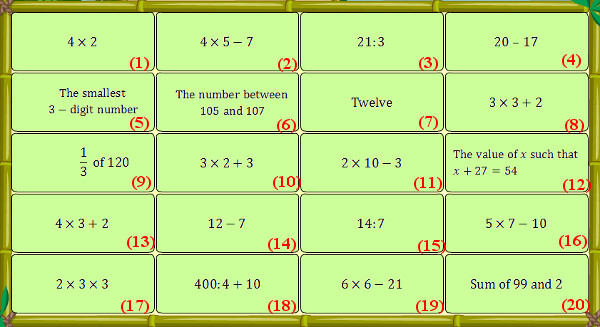Exam number 2: Fill in the blank with the suitable number

Question 1: Find x such that x + 34 = 99

Question 2: Write the missing number in the blank

Question 3: Calculate: 123 + 456

Question 4: Write in numbers "Nine hundred and twenty – two"

Question 5: Each box has 12 color pencils. How many pencils are in 3 of these boxes?

Question 6: Two apples weight 100 gram. Then ten apples weight ....gram.

Question 7: Write the missing number in the blank

Question 8: Write the missing number in the blank

Question 9: There are 56 students lining up in rows, there 7 students in each row. How many rows are there?

Question 10: The perimeter of rectangle ABCD is 20cm and the length of segment AB is 6cm. What is the length of segment BC?

Exam number 3: Vượt chướng ngại vật

Question 1: Which number makes the equation true?

163 + 100 = ........... + 102

a. 161           b. 262         c. 165           d. 365

Question 2: Find the value of x is ......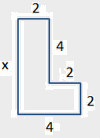a. 2            b. 8              c. 4            d. 6

Question 3: Which number is smallest?

 123 132 321 231

a. 321            b. 123             c. 231            d. 123

Question 4: What time will it be in 30 minutes?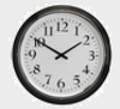a. 10:00          b. 2:20             c. 10:40            d. 1:20

Question 5: What is the perimeter?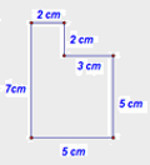a. 28cm          b. 25cm             c. 24cm            d. 49cm

Đáp án đề thi Violympic Toán tiếng Anh lớp 3 vòng 8

Exam number 1: Choose consecutively the cells with the increasing value to remove them from the table

(15) < (4) < (14) < (3) < (1) < (10) < (8) < (7) < (2) < (13) < (19) < (11) < (17) < (16) < (12) < (9) < (5) < (20) < (6) < (18)

Exam number 2: Fill in the blank with the suitable number

Question 1: 65        Question 2: 108      Question 3: 579

Question 4: 922        Question 5: 36       Question 6: 500

Question 7: 115        Question 8: 44       Question 9: 8         Question 10: 4

Exam number 3: Vượt chướng ngại vật

Question 1: a        Question 2: d           Question 3: b

Question 4: b        Question 5: c

Đề thi Violympic Toán Tiếng Anh lớp 3 vòng 7 năm 2015 - 2016

Exam number 1: Đi tìm kho báu

Question 1: Multiply 9 x 5 = .............

a. 48              b. 40              c. 47              d. 45

Question 2: Multiply: 9 x 7 = ...........

a. 62             b. 65              c. 61                 d. 63

Question 3: Multiply 9 x 2 = .............

a. 18            b. 14               c. 19            d. 16

Question 4: Multiply 9 x 9 = ..............

a. 81            b. 84                 c. 83          d. 82

Question 5: Calculate: 9 x 10 - 34 = .............

a. 65             b. 56               c. 64              d. 46

Question 6: .........g x 4 = 860g

The missing number is:

a. 205             b. 225              c. 215           d. 235

Question 7: ......... of the below circle is not colored.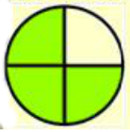a. 1/3               b. 1/5                c. 1/4              d. 1/6

Question 8: ........... of the figure below is colored.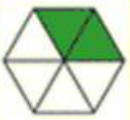a. 1/3              b. 1/5              c. 1/4          d. 1/6

Question 9: Mary has five packs of pencils. There are 9 pencils in each pack. John has 16 pencils. How many pencils do they have in all?

a. 51                b. 66             c. 56            d. 61

Question 10: The product of y and 9 is a two-digit number that has the digit 8 in the tens place. When y is divided by 9, the remainder is ................

a. 0                  b. 1           c. 9                d. 8

Exam number 2: Choose 2 consecutive cells which have the same or equal value.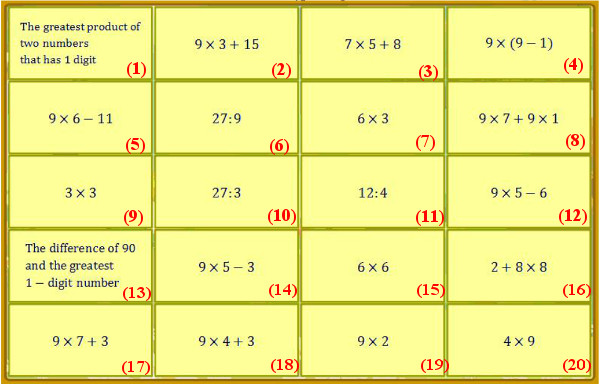Exam number 3: Fill in the blank with the suitable number

Question 1: 36 : .......... = 9

Question 2: 18 : 9 = .............

Question 3: .......... : 9 = 3

Question 4: 63 : 9 = ...............

Question 5: Follow the arrows and find the result: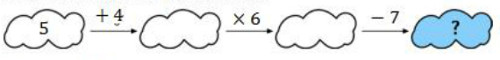Question 6: 1/5 of 45g is ................g.

Question 7: 1/N of 54g is 9g. The value of N is ................

Question 8: 1/M of 72g is 9g. The value of M is ..................

Question 9: The product of x and 9 is the greatest 2-digit number. The value of x is ...............

Question 10: Adding 35 to the smallest two-digit number and dividing the sum by the biggest one-digit number we get ..................

Đáp án đề thi Violympic Toán tiếng Anh lớp 3 vòng 7

Exam number 1: Đi tìm kho báu

Question 1: d        Question 2: d         Question 3: a

Question 4: a       Question 5: b         Question 6: c

Question 7: c       Question 8: a         Question 9: d          Question 10: c

Exam number 2: Choose 2 consecutive cells which have the same or equal value.

(1) = (13); (2) = (14); (3) = (5); (4) = (8); (6) = (11); (7) = (19); (9) = (10); (12) = (18); (15) = (20); (16) = (17)

Exam number 3: Fill in the blank with the suitable number

Question 1: 4        Question 2: 2         Question 3: 27

Question 4: 7        Question 5: 47        Question 6: 9

Question 7: 6        Question 8: 8         Question 9: 11         Question 10: 5

Đề thi Violympic Toán Tiếng Anh lớp 3 vòng 10 năm 2015 - 2016

Exam number 1: Điền giá trị thích hợp vào chỗ chấm

Question 1: Fill in the blank with the suitable sign (>; =; <)

Compare: 4782 .............. 4827

Fill in the blank with the suitable number

Question 2: 9 hours 5 minutes = ............ minutes

Question 3: 5km 12m = .............. m

Question 4: Calculate: 2088 : 6 = ................

Question 5: Alan is five years old. His sister Bethany is seven years older than him. What is the sum of their ages?

Question 6: Anna is reading a book that has 192 pages. Mary is reading a book that has three times as many pages as Anna's book. How many pages does Mary's book have?

Question 7: There are 3 bags of beans. Each bag contains 78 beans. How many beans are there in all?

Question 8: If 63 breads are divided to 7 students, how many breads will each student have?

Question 9: I am an odd 2-digit number. The sum of my digits is 9. My greatest value is ................

Question 10: If you add 10000 to the largest 5- distinct digit number that can be formed by using the digits 5, 7, 6, 4, 2, then what is the resulting sum?

Exam number 2: Đi tìm kho báu

Question 1: Calculate: (3726 + 2954) x 0 = ...........

Question 2: Find m such that 9384 : m = 4.

Question 3: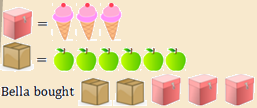She bought ...... less ice-creams than apples.

Question 4: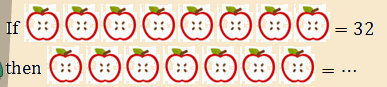Question 5: If the 2nd day of a month is Monday then the 27th day of the month is ........

a. Thursday

b. Friday

c. Wednesday

d. Tuesday

Exam number 3: Tìm cặp bằng nhau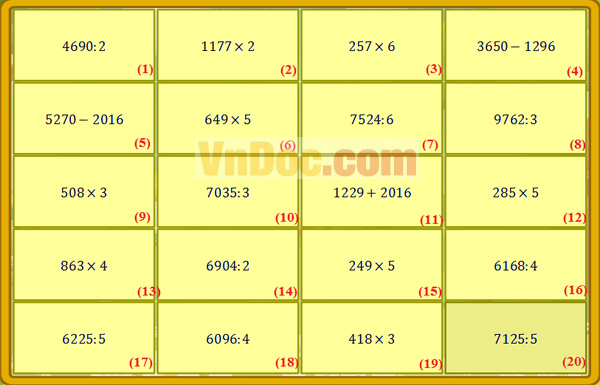Đáp án đề thi Violympic Toán Tiếng Anh lớp 3

Exam number 1: Điền giá trị thích hợp vào chỗ chấm

Question 1: <

Question 2: 545

Question 3: 5012

Question 4: 348

Question 5: 17

Question 6: 576

Question 7: 234

Question 8: 9

Question 9: 81

Question 10: 86542

Exam number 2: Đi tìm kho báu

Question 1: 0

Question 2: 2346

Question 3: 3

Question 4: 28

Question 5: b

Exam number 3: Tìm cặp bằng nhau

(1) = (10); (2) = (4); (3) = (16); (5) = (8); (6) = (11); (7) = (19); (9) = (18); (12) = (20); (13) = (14); (15) = (17)

Đề thi Violympic Toán Tiếng Anh lớp 3 vòng 9 năm 2015 - 2016

Exam number 1: Đỉnh núi trí tuệ

Question 1: Find x such that x : 6 = 42

a. 36            b. 7                 c. 48          d. 252

Question 2: Fill in the blank with a suitable operator 18 ........ 9 = 2

a. +             b. x                  c. :            d. -

Question 3: How many days are there in January?

a. 28            b. 29               c. 30           d. 31

Question 4: How long is segment AB in cm?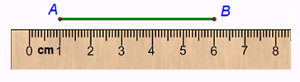a. 50              b. 60                c. 5                d. 6

Question 5: Find the value of x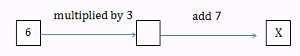a. 11               b. 9                 c. 126           d. 25

Question 6: Multiply 12 by 6 then subtract 25 from the product. The result is ............

a. 47                b. 27                c. 74             d. 97

Question 7: Divide 56 by 7 then subtract 5 from the quotient. The result is .............

a. 13                 b. 58              c. 3               d. 397

Question 8: By how many times is 15 greater than 5?

a. 10                 b. 20              c. 3                  d. 75

Question 9: Look at this table. Among these 4 people, who is the tallest?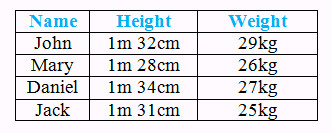a. Jack               b. John             c. Daniel           d. Mary

Question 10: Find the value of X: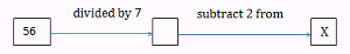a. 47                b. 10                 c. 6                 d. 65

Exam number 2: Cóc vàng tài ba

Question 1: There are 165 students in a grade 3, including 84 girls. How many boys are there in the grade 3?

a. 13860               b. 100                    c. 249            d. 81

Question 2: How do you write this number using digits? Seven hundred three.

a. 137                 b. 730                     c. 703            d. 173

Question 3: How many triangles are there?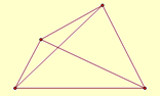a. 4                    b. 6                      c. 2             d. 8

Question 4: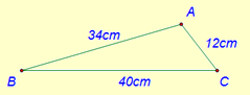The longest side of triangle ABC is ............ cm.

a. 40               b. 34                     c. 86           d. 12

Question 5: Danny is 31. Garrett is 4 years older than Bryce and 2 years older than Danny. How old is Bryce?

a. 33 years old                        b. 37 years old

c. 27 years old                        d. 29 years old

Question 6: Clayton is 5 years younger than Ruth. Jane is 36. Kimberly is 1 year younger than Jane and 5 years younger than Ruth. How old is Ruth?

a. 35 years old                         b. 37 years old

c. 41 years old                        d. 40 years old

Question 7: I am an even number. I am greater than 2 but less than 6. What number am I

a. 2                   b. 8                    c. 6              d. 4

Question 8: In a class, there are 40 students and the number of girls is 4 times the number of boys. How many boys are there?

a. 32                  b. 44                     c. 8               d. 10

Question 9: How many bricks like this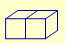are needed to make a wall like this?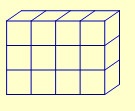a. 6                  b. 12                    c. 4                d. 3

Question 10: In my class, only 6 of 10 students were at school. How many were absent?

a. 7                  b. 10                     c. 6               d. 4

Exam number 3: Vượt chướng ngại vật

Question 1: How many green squares are there in the figure?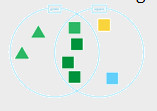Question 2: Coffee costs \$5 per pound. How much does 5 pounds of coffee cost?

Question 3: How many circles are there?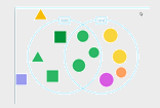Question 4: Look at the four angles marked on the picture of a house. Which angle is a right angle?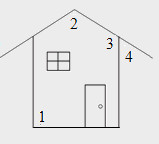Question 5: On Friday, 1250 people visited the zoo. The number of people visited the zoo on Saturday is three times as many as on Friday. How many people visited the zoo on Saturday?

Đáp án đề thi Violympic Toán Tiếng Anh lớp 3 vòng 9

Exam number 1: Đỉnh núi trí tuệ

Question 1: d

Question 2: c

Question 3: d

Question 4: c

Question 5: d

Question 6: a

Question 7: c

Question 8: c

Question 9: c

Question 10: c

Exam number 2: Cóc vàng tài ba

Question 1: d

Question 2: c

Question 3: d

Question 4: a

Question 5: d

Question 6: d

Question 7: d

Question 8: c

Question 9: a

Question 10: d

Exam number 3: Vượt chướng ngại vật

Question 1: 4

Question 2: 25

Question 3: 7

Question 4: 1

Question 5: 3750

Đánh giá bài viết
2 1.600